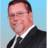Lookup table

Hi. Looking for help with a lookup table. I have two tables with three fields each. Table a has a.code, a.amount and a.tax; a.code and a.amount having values already.
I have lookup table b with fields b.code, b.amount and b.tax.
I would like to lookup a.code and a.amount in b.code and b.amount and return the value of b.tax to a.tax.
Any ideas?

Found a workaround :-)

What was the solution ?Not sure why you are using BOTH the code AND the amount.. but it could be something like..

`a.tax := first(select b [Code = a.code and Amount = a.amount])`

Thanks, Mconneen. It doesn't quite work, probably because I haven't referenced a record in b to a. This is what I want the query to do. I have hundreds of entries for each code, and each code has many amounts. The amounts are the same for all codes, but the third field, tax is different, and is the result and what I want to link the two tables by.

@Jon; I simply looked up b.tax (show as tax) from a and constrained the selection so that only one record (and the only right one)  is available to select . Constraints: a.code = b.code and a.amount= b.amount.

It works, but it would be much more elegant if the lookup was automatic.